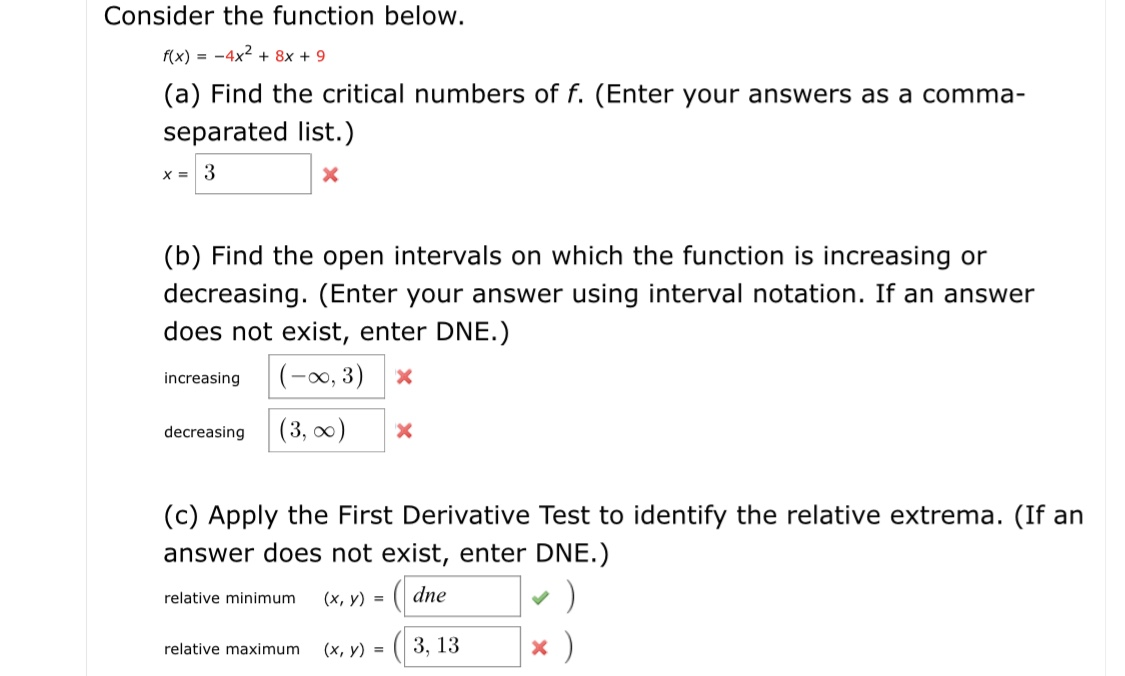# Consider the function below.f(x) = -4x2 + 8x + 9(a) Find the critical numbers of f. (Enter your answers as a comma-separated list.)(b) Find the open intervals on which the function is increasing ordecreasing. (Enter your answer using interval notation. If an answerdoes not exist, enter DNE.)(-0, 3)increasing(3, 0)decreasing(c) Apply the First Derivative Test to identify the relative extrema. (If ananswer does not exist, enter DNE.)dne(х, у) %3Drelative minimumх)3, 13(х, у) %3Drelative maximum

Question
82 viewshelp_outlineImage TranscriptioncloseConsider the function below. f(x) = -4x2 + 8x + 9 (a) Find the critical numbers of f. (Enter your answers as a comma- separated list.) (b) Find the open intervals on which the function is increasing or decreasing. (Enter your answer using interval notation. If an answer does not exist, enter DNE.) (-0, 3) increasing (3, 0) decreasing (c) Apply the First Derivative Test to identify the relative extrema. (If an answer does not exist, enter DNE.) dne (х, у) %3D relative minimum х) 3, 13 (х, у) %3D relative maximum fullscreen
check_circle

Step 1

Given,

Step 2

a). Critical numbers are the values of x for which first derivative is zero.

So, we find the derivative of f(x), that is

Step 3

b). A function increases if its first derivative is positive and ...

### Want to see the full answer?

See Solution

#### Want to see this answer and more?

Solutions are written by subject experts who are available 24/7. Questions are typically answered within 1 hour.*

See Solution
*Response times may vary by subject and question.
Tagged in
MathCalculus

### Derivative